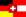Seite auf deutsch

# Quicksort

Quicksort was invented in 1962 by C.A.R. Hoare. It is the fastest comparison-based sorting algorithm in practice.

Legend:
pink: greater value in current comparison
red: smaller value in current comparison
yellow: pivot element
orange: the part that is already sorted
blue: animation running

The idea is the following:

1. If the array contains less than two items, we are finished.
2. Remove an (arbitrary) element p from the array R.
3. Then partition the array into two sub-arrays R1 and R2, with
R1 = {x in R | x<p} and R2 = {x in R | x>=p}
4. Each element in R2 is greater than the greatest element in R1. Thus we can sort the two subarrays independently. We start with each of the two subarrays at step 1.

When implementing this idea it is of central importance that the partitioning step is carried out efficiently. Another interesting point is the choice of the pivot element p. For simplicity, we take the rightmost item in this implementation. Quicksort performs best if the recursion tree is as well-balanced as possible. Then the running time (in O-notation) is O(n log n). In the worst case, however, the recursion tree is entirely unbalanced, and the running time becomes quadratic. Since we always choose the rightmost element as the pivot element, the worst-case input is an array that is entirely sorted.

If we use instead an implementation where the pivot element is chosen in each round at random, the expected running time will be in O(n log n). This expected running time holds on each input (in particular on worst-case inputs)1. Observe that in this case, we talk about a randomized algorithm, where the running time depends on the random choices. There the running time is a random variable, and thus we are interested in the expected value of the running time on a given input (e.g. a best-case, or worst-case input).

#### Footnotes:

1 Beware! The expected running time on worst-case input of a randomized algorithm is often confused with the running time of a non-randomized algorithm on average-case input. But the first concept is the expected value over the random choices the algorithm makes on a single input (only a single input involved, but many random choices). The second concept is the arithmetic mean of the running times on all possible inputs (many different inputs involved, but no randomness). By the way, for randomized algorithms, it also makes sense to speak about the expected average-case running time, but let not take the discussion too far...

#### References:

• C.A.R. Hoare: Quicksort. Computer Journal, Vol. 5, 1, 10-15 (1962)